### Agricultural Science Past Questions

2421

Study carefully the diagrams below marked D and E(a) (i) ldentify the farm implements D  and E as as illustrated by the diagrams.
(ii) Name the parts labelled I to VI.

(b) Describe briefly each of the implements illustrated by diagrams D and E

WAEC 1992
2422

(a) ldentify specimens F, G, H, and I.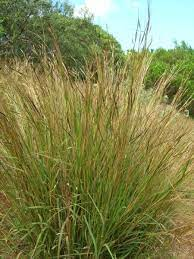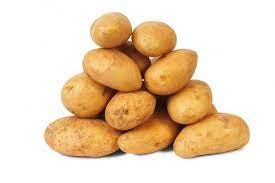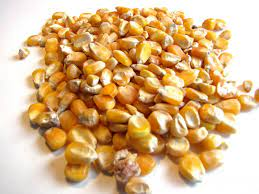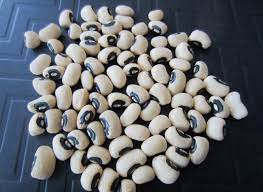(b) How is each specimen propagated?

(c) State two economic importance of each of specimens F, G and l and one economic importance of specimen H to man.

(d) Classify specimens F, G, H and l into annual and perennial crops.

WAEC 1992
2423

(a) ldenitity specimens J, K and L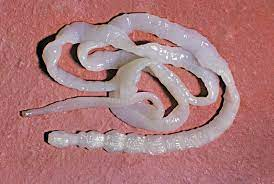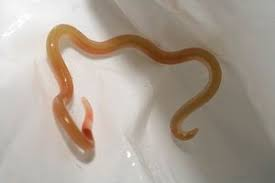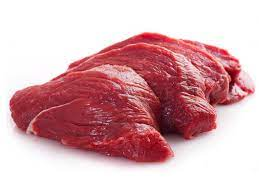(b)(i) Name two animal hosts for each of specimens J and K
(ii) In what part of the host is each of specimens J and K located?

(c) In a tabular forrn, state three differences between the eternal features of specimens J and K.

(d) Which of specimens J and K can be transmitted through specimen L to man?

(e) Name three intermediate hosts of specimen J.

WAEC 1992
2424

Study the diagrams below(a) ldentify the implements represented by diagrams l and ll above.

(b) List three important parts of each of the implements illustrated by diagrams I and lI

(c) State one function of each of the parts of the implements listed in (b)

(d) State:(i) one major advantage in using the implement illustrated by Il:
(ii) one major disadvantage in using the implement illustrated by I.

WAEC 1991
2425

The diagram below illustrates the experimental setup used to determine soil texture.(a) Name the parts labelled A to F on the diagram.

(b) List four major constituents of the soil

(c) State three other methods which can be used to determine soil texture

(d) Explain briefly two importance of soil texture to the farmer

WAEC 1991